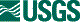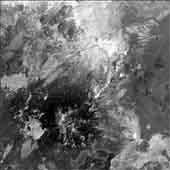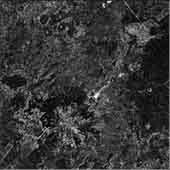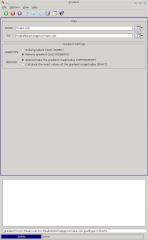## ISIS 2ISIS Application Documentation

Printer Friendly View | TOC | Home

Apply Sobel or Roberts gradient to a cube

 Overview Parameters Example 1 Example 2

## Description

This program will apply a Sobel or Roberts gradient filter to a cube. These gradient filters will highlight edges of an image. Gradient filters typically are used to highlight the differences in the brightness of adjacent pixels in a cube, and also the edges of the transitions in the image data. The output will contain the calculated magnitude of the spatial graident of the input cube. The calculation method can be set to either exact or approximate and can be specified with the 'METHOD' parameter. NOTE: When a calculation must be done where one or more values are special pixels, the output will be NULL. If this causes unexpected results, you can run stretch to change ithe input special pixel(s) to a Digital Number (DN) that is appropriate for calculation. For example, if the image contains High Instrument Saturation (HIS) values, you may choose to stretch these to 255 prior to running the gradient filter.

## History

 Tracie Sucharski 2002-12-18 Original version Kim Sides 2003-05-13 Added application test Stuart Sides 2003-05-16 Modified schema location from astogeology... to isis.astrogeology..." Stuart Sides 2003-07-29 Modified filename parameters to be cube parameters where necessary Drew Davidson 2005-08-16 Added examples Brendan George 2005-11-07 Modified to handle Special Pixels Ian Humphrey 2015-05-18 Added METHOD parameter to allow for either exact or approximate calculation of the gradient magnitude. Updated and added tests. Updated documentation. Fixes #1741.

## Parameter Groups

### Files

Name Description
FROM Input cube
TO Output cube

Name Description
METHODMethod for calculating the gradient (Approximate, Exact)

### Files: FROM

#### Description

Use this parameter to select the filename. All bands within the file will have the gradient applied.

 Type cube input *.cub

### Files: TO

#### Description

This file will contain the estimated or exact absolute magnitude of the gradient.

 Type cube output real

#### Description

This chooses the gradient type. The possibilities are Sobel, which uses a 3 x 3 boxcar to calculate the gradient magnitude, or Roberts, which uses a 2 x 2 boxcar to calculate the gradient magnitudes.

Type string
Default SOBEL
Option List:
Option Brief Description
SOBELSobel gradient (3x3) The Sobel gradient uses a 3 x 3 boxcar that makes the gradient calculation slow but less susceptible to noise. It will also create higher output for edges than the Roberts gradient.
ROBERTSRoberts gradient (2x2) The Roberts gradient uses a 2 x 2 boxcar that makes the gradient caluclation fast but sensitive to noise. It also works best on sharp, obvious edges.

#### Description

This specifies how the gradient magnitudes are calculated. The possibilities are exact, which uses the square root of the sum of squares, or approximate, which uses the sum of absolutes.

Type string
Default APPROXIMATE
Option List:
Option Brief Description
APPROXIMATEApproximate the gradient magnitudes An approximate calculation can be achieved using the sum of absolute values of the gradient components. This method is a bit faster than the exact calculation method. The approximate gradient magnitude is calculated as follows:
```              G = |Gx| + |Gy|
```
EXACTCalculate the exact values of the gradient magnitudes An exact calculation can be achieved using the square root of the sum of the squares of the gradient components. This method is slower than the approximate calculation method. The exact gradient magnitude is calculated as follows:
```              G = sqrt(Gx2 + Gy2)
```

### Example 1

Applying a sobel filter

#### Description

The use of the Sobel gradient here creates an output image which helps to highlight the edges.

#### Command Line

This example shows the use of the sobel filter in order to highlight edges

#### GUI Screenshotgradient gui Example GUI Screenshot of the GUI with parameters set to filter using a sobel configuration

#### Input ImageThe image before the filter Input image before the filter. Parameter Name: FROM This is the image as it was taken originally.

#### Output ImageThe image after the filter Output image after the gradient was applied Parameter Name: TO As you can see, sharp edges on the image have been emphasized, while areas of more constant DNs have been de-emphasized.

### Example 2

Applying a roberts filter

#### Command Linegradient gui Example GUI Screenshot of the GUI with parameters set to filter using a roberts gradientThe image before the filter Input image before the filter. Parameter Name: FROM This is the image as it was taken originally.The image after the filter Output image after the roberts gradient Parameter Name: TO The use of the Roberts gradient here creates an output image which helps to highlight the edges. Since the Roberts filter uses a smaller boxcar, less of the image is taken into consideration at each processing step and thus the image can be created faster. The tradeoff is that images that have had the Roberts gradient applied tend be effected more by noise in the image.